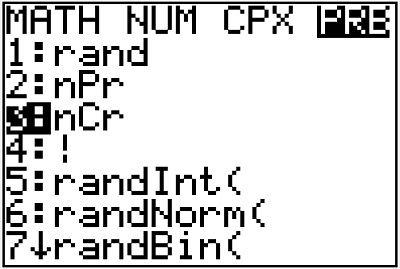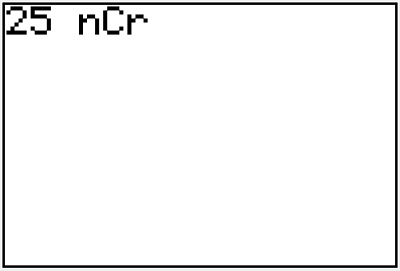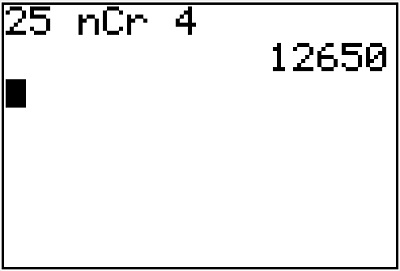# Combinations on the TI83 or TI84 calculator

In counting, combinations are used to find the number of ways a selection can be made, when order doesn’t matter. In this article, we will see how to use a calculator to find combinations. Let’s use an example to see how this works!

You are taking a week-long trip and decide to bring 4 books from your collection of 25 books. How many different groups of 4 books can be selected from your collection?

Here, you only care about which four books are chosen, not the order. Thus, a combination can be used to answer this question: C(25, 4) or “25 choose 4”.

## Step 1: Type in the first number

In this case, the first number is 25.## Step 2: Press [MATH] and go to the PRB menu

You can use the right arrow to select the menu at the top.## Step 3: Select 3 nCr and press [ENTER]

To select 3: nCr, you can either highlight the 3 or just press the 3 button.## Step 4: Type the second number and press [ENTER]

We are finding C(25,4), so the second number is 4.From this, we see that there are C(25, 4) = 12,650 different groups of 4 books that could be selected. This may seem like the answer is too large, but if you start thinking about how only one book needs to be different for the group of 4 books to be considered a different group, it begins to make more sense.

## Other methods for calculating combinations

You can also use the formula to calculate a combination. You can review this, and more about combinations in general, below.

Counting with combinations